## Math 480 Course Notes -- June 4, 1996

### Solution of linear diffrential equations

Consider the linear differential equation of the form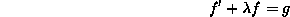where f and g are functions of t and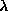is a constant. We assume that g(t) is given and we wish to find f(t).

To solve such equations, we make the left side have the form of the derivative of a product by multiplying through by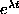: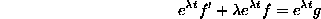We recognize the left as the derivative of the product ofand f: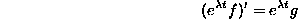We can integrate both sides of this (with s substituted for t) from 0 to t to recover f(t):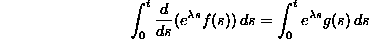Of course, the integral of the derivative on the left side of the equation may be evaluated using the (second) fundamental theorem of calculus: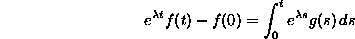Now we can solve algebraically for f(t):Dennis DeTurck
Fri Jun 7 16:34:24 EDT 1996